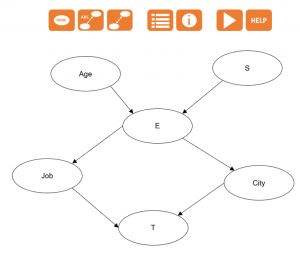# Bayesian networks

Use this tool to model dependency or conditional independence relationships between random variables.## What is a Bayesian network?

A Bayesian network is a statistical tool that allows to model dependency or conditional independence relationships between random variables. This method emerged from Judea Pearl’s pioneering research in 1988 on the development of artificial intelligence techniques.

The originality of this method is that it offers a formal framework to represent the relational structure of network variables. On one side we have a qualitative description with a graph and on the other side a quantitative description with probabilities. The graph is a way to schematize the network with nodes and arcs connecting these nodes, which will facilitate understanding of the problem and interpretation of the results.

Bayesian networks are used in the fields of finance, medicine or industry to model and analyze risks of credit card fraud for example or to help the medical profession make a diagnosis.

## Analyzing a Bayesian network in XLSTAT

The procedure for analyzing a Bayesian network in XLSTAT is as follows:

### A. Open a project

In the XLSTAT menu go to the Bayesian Networks module and open a new project. A window opens offering the choice between classic and expert mode. A raw Bayesian Networks project always initially contains two sheets and then three when the probability tables are defined. These sheets, which should not be deleted, are:

• Data: it is an empty Excel sheet in which your data must be copied/pasted.
• BNGraph: it is a sheet containing a drawing area, blank at the beginning, and a toolbar whose description is made in the next section. It must be used to draw the graph of the Bayesian network.
• Probabilitytables: this is a sheet that contains the probability distributions of all nodes that were drawn on the BNgraph sheet.

### B. Construct the graph

Draw your network using the provided buttons and name each of your nodes.

The graph of a Bayesian network is materialized by nodes and arcs that link nodes. In a project Bayesian networks, the graph must be drawn on the sheet named BNGraph with the toolbar. The selection of nodes and arcs is done using the toolbar available at the top left of the BNGraph sheet which includes height orange buttons aligned horizontally:The first four buttons are dedicated to the drawing of the Bayesian network, the next two to fill in the probability table, the seventh is used to compute the probabilities of the Bayesian network drawn and the last button redirects to this help.

### C. Definition of probability distributions

For each variable you must fill in a probability table. This can be done in two ways: expert mode, that is, from human knowledge or learning mode, that is, the Bayesian network feature can be learned automatically from data.

### D. Inference

Once the model is designed on the BNGraph sheet, and once all the probabilities have been defined for each variable, you can click on the button Run of the toolbar to launch the analyses on the Bayesian network.

## Results of the XLSTAT functionality Bayesian network

The results obtained answer all possible queries on the Bayesian network analyzed.

Marginal probability distribution of each node: this result, given as a table, corresponds to the marginal probabilities of each node drawn on the BNGraph sheet. If the graph option is selected the result is also displayed as a bar chart under the table.

Join probability distribution for each clique: This result displays the number of cliques, the list of nodes involved in each of cliques and their correlated join probability distribution table.

Conditional probability distribution of each node: this result, given as a table, corresponds to the conditional probabilities of each dependent node. If the graph option is selected the result is also displayed as a bar chart under each table.### analyze your data with xlstat

14-day free trial

Included in